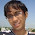### Expected number of draws

Source: http://deltaepsilons.wordpress.com/

Problem: Consider a set of n objects from which m are drawn randomly at a time, with replacement.  What is E(n,m), the expected number of draws I have to make to have drawn all of the objects?

Note that we solved a similar problem and got the value of E(n,1) some time back in this problem.

1.found an approximate bound using wald's equation. http://en.wikipedia.org/wiki/Wald's_equation.

imagine the experiment with m=1.its known that
E(n,1)= n H(n),
where H(n)=1+1/2+1/3+....1/n

now let x1= number of draws to pick m distinct objects. After x1 draws, let x2=number of draws to pick m distinct objects, and so on till u see see all the n objects.

then E[X1]=E[X2]=...=n[1/(n)+1/(n-1)+...1/(n-m+1)]=n/[H(n)-H(n-m)]

If S is total number of draws
i.e. S=X1+X2+.......,then as per wald's equation,
E[S]=E[X1]*E(n,m).

Now, E[S]-m < E(n,1) <=E[S].

Inequality is there because last object will be drawn in the last set of m draws.so,

[H(n)+m/n]/[H(n)-H(n-m)] > E(n,m) > = H(n)/[H(n)-H(n-m)]

where H(r)=1/r+ 1/(r-1)+..1/2+1

2.A small corection.

i wrote in previous post that
E[S]-m < E(n,1) <= E[S].

this needs to be changed to
E[S-X] < E(n,1) <= E[S].

where X is the number of draws required to pick the last group of m distinct objects. The reason is that by definition, the S draws cover all n objects and first S-X draws do not cover all n objects.

With this correction,we get

A+1 > E(n,m) >= A

where A= H(n)/[H(n)-H(n-m)]

and H(r)=1/r+ 1/(r-1)+..1/2+1.

3.Not entirely sure if this solution is correct - do comment :)

Consider a bit-string of length n. 1 denotes the corresponding object has already been selected, 0 denotes that it has not been selected. We need to find the expected number of turns needed for all the bits to become 1, which would be the same as the expected number of turns needed for any individual bit to become a 1.

The total number of ways in which we can have m 1's in the bit string is nCm.
Consider the i-th bit. Number of ways in which this bit is 1 is (n-1)C(m-1).
So probability that the i-th bit is 1, P(i) = (n-1)C(m-1) / nCm = m/n
If N is the expected number of turns needed for the i-th bit to be 1, N * P(i) = 1, so N = n/m
This is the same for any bit. So expected number of moves is n/m.

4.Sorry... the solution I posted is grossly wrong...

5.Consider the stage when i of the n objects have turned up. The probability that in the next draw we shall get one of the remaining (n-i) objects is (n-i)Cm/ nCm. Hence the expected number of steps required to get to get one of these (n-i) objetcs = nCm/(n-i)Cm [geometric distribution]
So, expected number of steps required to get all objects = sum(i=1 to n-m) of [nCm/(n-i)Cm]

6.Can anybody tell me how to solve this? I do not understand chera's solution well.